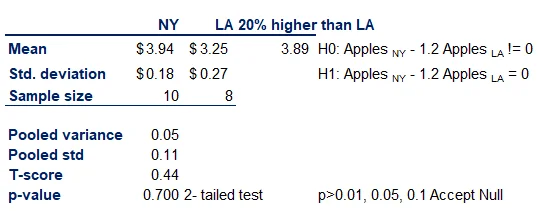12 Jan 2023

Posted on:

12 Sept 2021

1

# Where can I find the solution for the homework portion?

The values I used/got are as follows. Can you please tell me whether they are correct if you have the time? I would highly appreciate it.

I was a bit slow to grasp all the concepts and patterns and I think I am finally getting the hang of it. An answer at this moment in time would be golden to me.

Thank you very much.

Null hypothesis NY apples are not exactly 20% more expensive than LA apples.
Hypothesis value \$ 3.89
T score 0.81
p value 0.439
At 10% significance Accept null hypothesis
At 5% significance Accept null hypothesis
At 1% significance Accept null hypothesis
Conclusion NY apple are not exactly 20% more expensive than LA apples.
Posted on:

13 Sept 2021

5

Hello Sachintha!

Thanks for reaching out!

I would suggest trying a different approach to avoid the ambiguity of using "not" in any hypothesis statement.

We can hypothesize that the apples in NY are at least \$0.65 higher than those in LA. (Put in a different way they are 20% higher).

In that case the T-score would be equal to \$3.94-\$3.25-\$0.65 divided by the pooled standard deviation. Put simply the result would be 0.44

So, you cannot reject the null hypothesis. They are indeed at least \$0.65 higher.

The difference in this approach is that we used a precise value that you can test.

Best,
The 365 Team

Posted on:

13 Sept 2021

0

I thought about your hypothesis too and I spent a lot of time juggling the two before I settled on mine. Thank you very much for your quick answer. Your approach to the hypothesis would be very helpful in the future.

P.S. - The lessons are awesome by the way. Thanks for your efforts.

Posted on:

13 Sept 2021

0

Appreciate it!

Keep on the good progress!

Best,
The 365 Team

Posted on:

02 Dec 2022

0

This reply is meant for Ivan Kitov.

Hi, Ivan. I have a doubt regarding the null hypothesis that you stated. From the video, I would have thought that the null hypothesis was if the prices of apples in NY are EXACTLY 20% higher than those in LA, and that's because I didn't see the words "at least." Can you help explain this?

Julián

Posted on:

12 Jan 2023

0

Dear Instructor,
I would like to approach the topic differently. The question is "Is the price of Apples in NY 20% higher than in LA?". In my opinion, the H0 and H1 need to be as follows:
H0: (Apples NY - 1.2 Apples LA) != 0; H1: (Apples NY - 1.2 Apples LA) = 0. Proceeding further, please find the working below. Can you please verify whether this approach is correct because it differs from the one defined by the instructor in one of the above replies? Though the decision remains same, I have considered this a 2-tailed test rather than 1-tailed. Also, a positive T-score shows that the difference is > 20%